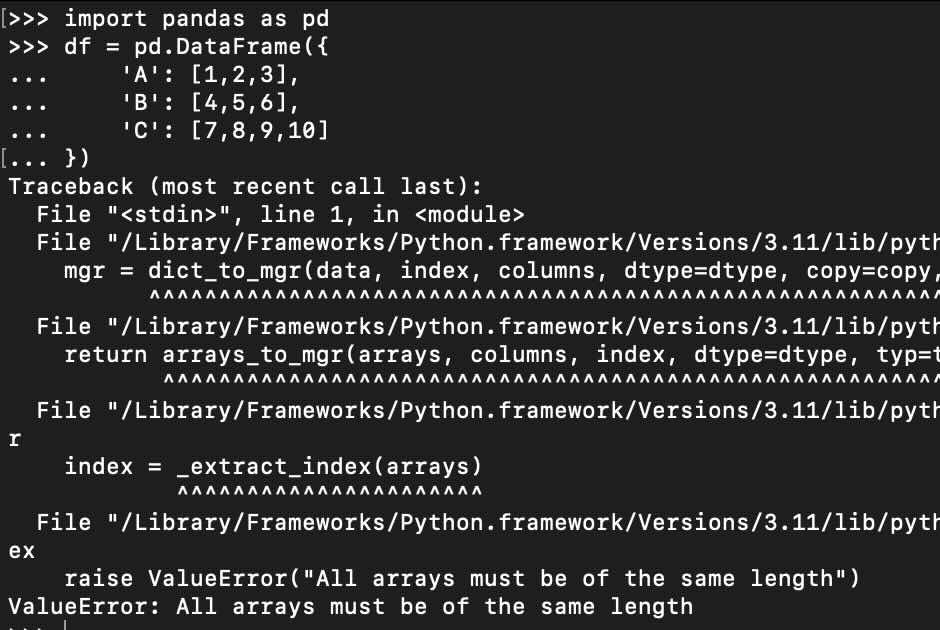# [Python] Fix: ValueError: All arrays must be of the same length

``````>>> import pandas as pd
>>> df = pd.DataFrame({
...     'A': [1,2,3],
...     'B': [4,5,6],
...     'C': [7,8,9,10]
... })

Traceback (most recent call last):
File "<stdin>", line 1, in <module>
File "/Library/Frameworks/Python.framework/Versions/3.11/lib/python3.11/site-packages/pandas/core/frame.py", line 709, in __init__
mgr = dict_to_mgr(data, index, columns, dtype=dtype, copy=copy, typ=manager)
^^^^^^^^^^^^^^^^^^^^^^^^^^^^^^^^^^^^^^^^^^^^^^^^^^^^^^^^^^^^^^^^^^^^^^
...
...

raise ValueError("All arrays must be of the same length")
ValueError: All arrays must be of the same length``````

You will get an ValueError when working with Pandas DataFrame in Python if you create columns with different lengths.

All columns in a DataFrame must have the same length in Pandas.

As you can see in the above example, column C has a length of 4 whereas other columns have a length of 3.

Fix:

We make sure that the length of columns in the data frame are the same.

``````
df = pd.DataFrame({
'A': [1,2,3],
'B': [4,5,6],
'C': [7,8,9]
})``````-

### Facing issues? Have Questions? Post them here! I am happy to answer!Author Info:

Rakesh is a seasoned developer with over 10 years of experience in web and app development, and a deep knowledge of operating systems. Author of insightful How-To articles for Code2care.